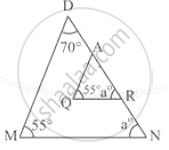# In the Following Figure, State Whether the Triangles Are Similar. Give Reason. - Geometry

In the following figure, state whether the triangles are similar. Give reason.#### Solution

Yes, the triangles are similar because of the Angle-angel -angle similarity.

M = Q = (each angle is 55°)

N = R = (each angle is a°)

Due to AA similar, these two triangles are similar.

Concept: Similar Triangles
Is there an error in this question or solution?